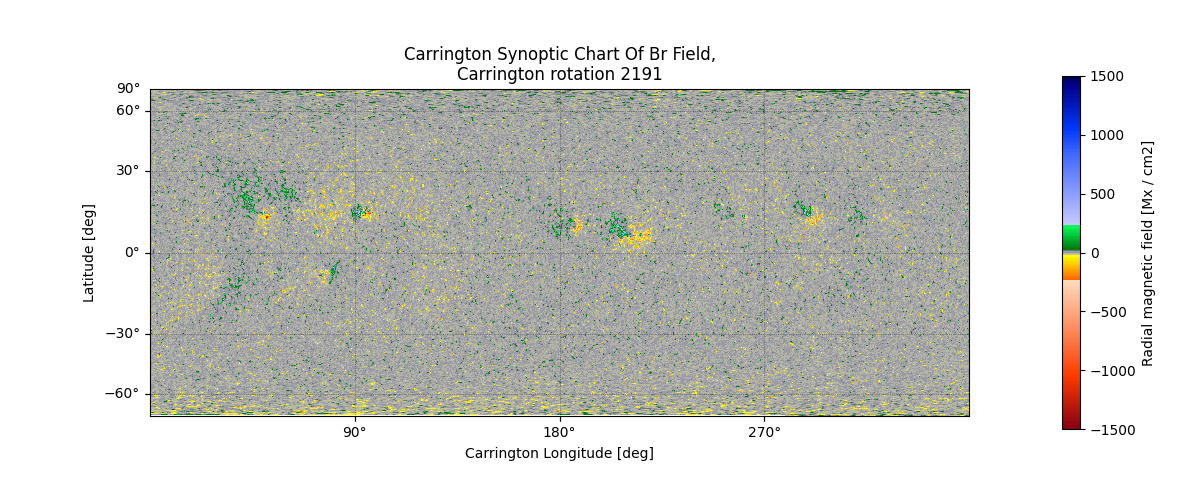In this example we load a synoptic map produced by the HMI team. This data is an interesting demonstration of sunpy’s Map class as it is not in the more common Helioprojective coordinate system, but in heliographic Carrington coordinates and a cylindrical equal area (CEA) projection.

```import matplotlib.pyplot as plt

import sunpy.map
```

```filename = download_file(
'http://jsoc.stanford.edu/data/hmi/synoptic/hmi.Synoptic_Mr.2191.fits', cache=True)
syn_map = sunpy.map.Map(filename)
```

Plot the results.

```fig = plt.figure(figsize=(12, 5))
ax = plt.subplot(projection=syn_map)
im = syn_map.plot(axes=ax)

ax.coords.set_axislabel("Carrington Longitude [deg]")
ax.coords.set_axislabel("Latitude [deg]")

ax.coords.grid(color='black', alpha=0.6, linestyle='dotted', linewidth=0.5)

# In order to make the x-axis ticks show, the bottom y-limit has to be adjusted slightly
ax.set_ylim(bottom=0)
ax.set_title(f"{syn_map.meta['content']},\n"
f"Carrington rotation {syn_map.meta['CAR_ROT']}")

plt.show()
``````INFO: Missing metadata for solar radius: assuming the standard radius of the photosphere. [sunpy.map.mapbase]
```

Total running time of the script: ( 0 minutes 2.445 seconds)

Gallery generated by Sphinx-Gallery